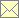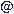Laser Doppler Data Processing Techniques - Forward-Backward (Inter-)Arrival-Time Weighting

 Home Scope Literature Data Programs Techniques Links Impressum Data Protection Policy Data Basewebmasternambisde 12.3.2018

If the inter-arrival times as used for the calculation of the mean or the variance ($w i = t i - t i-1$) are used as weighting factors for the estimation of the correlation function or that of the power spectral density, there will be a correlation between the respective time lags in the corresponding correlation function and the inter-arrival times. The reason for that is that a cross-product of velocities contributing to the correlation function requires that the two samples have exactly the respective inter-arrival time. If $u i$ and $u j$ are two velocity samples at times $t i$ and $t j$, where $t i < t j$, then the inter-arrival weight of the second sample $w j = t j - t j-1$ interferes with the time lag $t j - t i$ of the correlation function. Therefore, the usual inter-arrival times cannot be used directly as weighting factors for the correlation or the spectrum estimation. Instead, a cross-product of two velocity samples $u i$ and $u j$ can use the two inter-arrival weights

where $u i$ is assumed to be the first and $u j$ is the second sample ($t i < t j$). In that case, the arrival times are ordered as $t i-1 < t i < t j < t j+1$, and the two weights are independent of the inter-arrival time $t j - t i$ of the two samples.

However, also here, this weighting scheme works efficiently only at high enough data densities of the order of ten samples per integral timescale or larger. Therefore, Transit-Time Weighting should generally be preferred.

original papers (combined with local normalization and fuzzy slotting):

adapted to the direct spectral estimation: